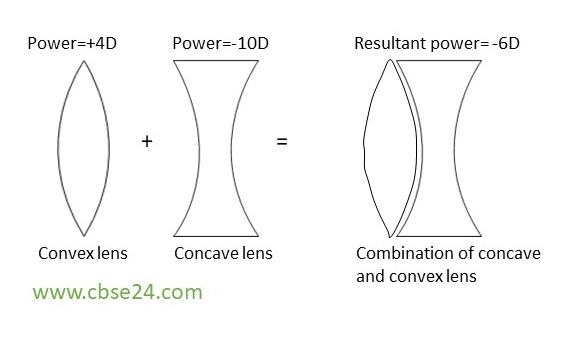# Power of lens- Definition,Unit (Diopter) ,Formula class 10 | cbse24

The power of a lens is a measure of the degree of convergence or divergence of light rays falling on it.

The power of a lens is defined as the reciprocal of its focal length in meters.

Poweof lens=1focalengtof lens(m)

P=1f(meters)

P=100  f(cm)

Note:-

• Unit of power of lens in Dioptre(D)
• The power of concave lens is negative(-ve)
• The power of convex lens is positive(+ve)
• A thick lens has short focal length but more power. A thin lens has large focal length but less power.

## Power of a combination of lenses:

If a number of lenses are placed in close contact, then the power of the combination of lenses is equal to the algebraic sum of the powers of individual lenses.

P=p1+p2

Example: Suppose convex lens has power +4D and concave lens of power -10D,So resultant power should be (4+(-10)=-6D) 6D. So this combination look like.

P= +4+(-10)
= +4-10
=  -6 DPower of a combination of lens

*